American Journal of Optics and Photonics
Volume 3, Issue 1, February 2015, Pages: 1-4

Parametric Characterization of Truncated Circular Flattened Gaussian Beams

Redouane Lamsoudi, Mohammed Ibn Chaikh

Laboratory of Physics of Condensed Matter, Physics Department, Faculty of Science, Chouaïb Doukkali University, El Jadida, Morocco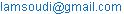(R. Lamsoudi)

Redouane Lamsoudi, Mohammed Ibn Chaikh. Parametric Characterization of Truncated Circular Flattened Gaussian Beams. American Journal of Optics and Photonic. Vol. 3, No. 1, 2015, pp. 1-4. doi: 10.11648/j.ajop.20150301.11

Abstract: Based on the truncated second-order moments definition, the generalized factor of the circular flattened Gaussian beams (CFGB) in the cylindrical coordinate system through a hard-edged circular aperture is derived. Three special cases have been obtained from the closed-form expression for the generalized factor of the truncated CFGB, the non-truncated CFGB, the truncated and non-truncated Gaussian beams. The power fraction of the CFGB is calculated analytically and illustrated numerically.

Keywords: Generalized Factor, Power Fraction, Circular Flattened Gaussian Beams

Contents

1. Introduction

In many applications, such as material thermal processing, inertial confinement fusion, second-harmonic generation, electron acceleration, and optical communication, a laser beam with a flat-topped spatial profile is required [1,2]. This allows one to make better use of all the energy that is present in the laser beam or to make use of the entire volume. Several theoretical models have been proposed to describe a coherent flat-topped beam of circular symmetry [3,4]. The desired uniform intensity distribution leads one to the study of Flattened Gaussian Beams (FGBs) or Super Gaussian Beams (SGBs). Flat-top laser beams can be realized by converting a Gaussian beam from a single transverse mode laser using an optical beam shaper . Several approaches have been reported for the design of flat-top beam shaping devices. The most straightforward method is to truncate or attenuate the input Gaussian beam using a neutral density filter with a proper transversal transmittance profile . The drawback of this approach is its poor energy efficiency. To improve the beam shaping efficiency, both reflective and refractive optical systems have been considered, resulting in the requirement of sophisticated optical surfaces that are difficult to fabricate and high beam shaper fabrication costs .

Recently, the characterization of laser beams has been the subject of many research works [7, 8]. In the last decade, many parameters and factors have been introduced in literature to describe different laser beams in practice. Among those parameters, we found the power in bucket (PIB), the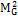factor which is used to predict the laser beam quality [9, 10]

The analytical calculation of the power entering through an aperture (the power fraction) and the generalized beam propagation factor for different laser beams rectangular symmetry has been based on the definition of the moments of intensity of order 2. However, we must appeal to the definition of moment of intensity truncated  when the ABCD optical system has an opening. In the literature, the laser beams truncated Gauss beams, cosh-Gauss beams and Hermite-cosh-Gaussian beams admit analytical solutions [12,13].

In this step of work, we determine the closed-form expression for the power fraction (PIB) and the generalized beam propagation factor for truncated circular flattened Gaussian beams. These two last parameters are already determined in an approximate tone . The truncated and no truncated Gaussian beams can be deduced as a particular case of the considered beams when N = 1.

2. Power Fraction of Circular Flattened Gaussian Beams

The electric field of circular flattened Gaussian beams at   z = 0 can be expressed as the following finite sum of Gaussian modes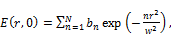(1)

Here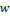is the waist width of the circular flattened Gaussian beams, the amplitude parameter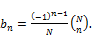(2)

Within Eq.(2),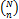denotes a binomial coefficient and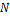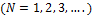is the order of the flattened Gaussian beams. When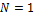, Eq.(1) reduces to a Gaussian beam.

The power fraction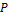is given as: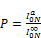(3a)

Where, the total power entering through the aperture of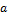radius is defined by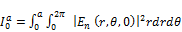.            (3b)

And, the total power of the beam through out the space.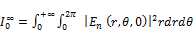.             (3c)

The substitution from Eq. (1) into Eq. (3b) and after some algebraic manipulation, we obtain the expression of the total power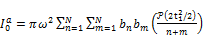(4)

Where, the probability integral of the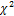-distribution defined by [15,16]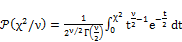(5a)

And(5b)

The truncation parameter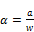(5c)

By letting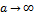and, use of the property of the probability function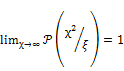(6)

One obtains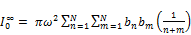(7)

Finally, Eq. (3a) can be written as(8)

This equation is a closed-form of the power fraction of circular flattened Gaussian beams. To illustrate this result.Fig.1 describes the variation ofversus truncation parameter α for some values of.

From this formula, we can deduce two limiting cases:

Ÿ for an infinitely large aperture. in this case the Eq.(8) is reduced to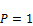(9)

Ÿ When N=1 the expression of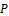reduced to the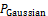.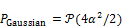(10)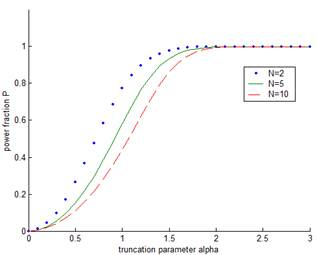Fig. 1. The power fractionas a function of truncation parameterfor various order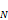.

3.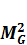-Factor of Truncated Circular Flattened Gaussian Beams

Following the Refs , we define the second-order irradiance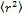in spatial domain,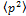in frequency domain and the cross second moment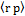of a hard-edged diffracted read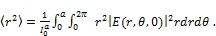(11)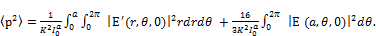(12)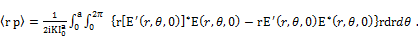(13)

The prime indicate derivation with respect to,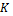is the wave number, and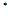is the complex conjugate.

The generalized beam propagation factor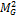-factor reads in the cylindrical coordinate system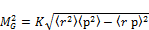(14)

Substituting from Eq. (1) into Eq. (11) can be written as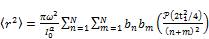(15)

Substituting from Eq. (4) in to Eq. (12) can be written as(16)

Where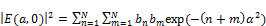(17)

Note that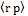vanishes since the Circular Flattened Gaussian field distribution is real valued in the waist plane.

Finally, the analytical expression of-factor can be written as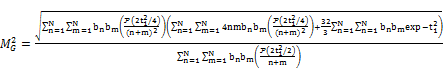(18)

From this formula, we can deduce two limiting cases:

Ÿ for an infinitely large aperture, in this case the Eq.(18) is reduced to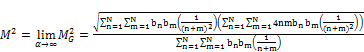(19)

Ÿ If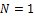corresponding to Gaussian beams. Using the relation between the probability integral of the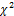-distribution and the incomplete gamma function, and the recurrence relation for the incomplete gamma function Eq.(18) simplifies to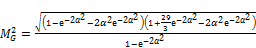(20)

The expression (18) is a closed-form of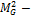factor of the circular flattened Gaussian beam passing through a hard-edged aperture. To illustrate Eq.(18), we present in Figs. 2 , thefactor versus the truncation parameter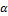for which the power fraction, for various values of.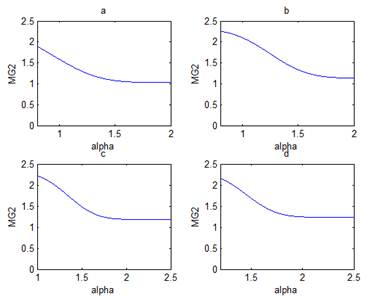Fig. 2.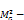factor of a circular flattened Gaussian beams versuswith (a)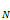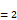, (b)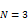, (c)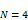, (d)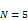.

These figures show that for all values of beam order, thefactor decreases with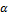and tends to the no truncated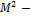factor. So, for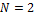, the value of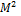is 1.0307 . From these figures, we can determine the values ofwhich are respectively: 1.1353, 1.1833, 1.2370 for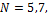and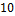. Also, we can determine from our analytical expression of thefactor some particular cases.

4. Conclusion

On the basis of the generalized truncated second-order moments, generalizedfactor of hard-edge diffracted circular flattened Gaussian is derived. The closed-form expression for thefactor of hard-edge diffracted CFGB is dependent on the truncation parameterand beam order N. Two special cases of M -factor of hard-edge diffracted CFGB, one is the case for→∞, for untruncated case, and the other is the case for N=1, for truncated Gaussian beam, have been discussed. In addition, the power fraction is demonstrated analytically and numerically.

References

1. N. Nishi, T. Jitsuno, K. Tsubakimoto, S. Matsuoka, N. Miyanaga, M. Nakatsuka, Opt. Rev.7 (2000) 216.
2. Y. Kato, K. Mima, N. Miyanaga, S. Arinaga, Y. Kitagawa, M. Nakatsuka, C. Yamanaka, Phys. Rev. Lett.53 (1984) 1057.
3. M.S. Bowers, Opt. Lett.19 (1992) 1319.
4. Y. Li, Opt. Lett.27 (2002) 1007.
5. J. Yang. R. Wang Opt. Eng. 42 ( 2003) 3106.
6. D. Shealy. SPIE.4095 (2000) .1
7. R. Martinez-Herrero, P.M. Mejias, Opt. Lett. 18 (1993) 1669.
8. R. Martinez-Herrero, P.M. Mejias, M. Arias, Opt. Lett. 20 (1995) 124.
9. A.E. Siegman, Proc. SPIE 1224 (1990) 2.
10. P.M. Mejías, H. Weber, R. Martínez-Herrero, A. González-Ureña (Eds.), Proceeding of Laser Beam Characterization, Seceded Espanda Optica, Madrid, Spain, 1993.
11. A.E. Siegman, IEEE J. Quant.Electron. 27 (1991) 1146.
12. B. Lu ¨, S. Luo, Opt. Commun. 178 (2000) 275.
13. M. Ibnchaikh, L. Dalil-Essakali, Z. Hricha, A. Belafhal, Opt. Commun. 190 (2001) 29.
14. Z. Mei, D. Zhao .APPLIED OPTICS. 44 (2005) 1383.
15. M. Abramowitch, I. A. Stegun (Eds), Handbook of Mathematical Function, Nath Bureau of Standards, Washington, DC (1964).
16. .S. Gradshteyn, I.M. Ryznik, Tables of Integrals, Series, and Products, fifth ed., Academic Press, New York, 1994.

 Contents 1. 2. 4.
Article ToolsAbstractPDF(209K)